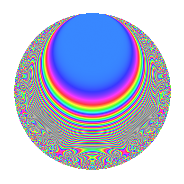# Properties

 Label 804.2.e.bLevel 804 Weight 2 Character orbit 804.e Analytic conductor 6.420 Analytic rank 0 Dimension 34 CM No

# Related objects

## Newspace parameters

 Level: $$N$$ = $$804 = 2^{2} \cdot 3 \cdot 67$$ Weight: $$k$$ = $$2$$ Character orbit: $$[\chi]$$ = 804.e (of order $$2$$ and degree $$1$$)

## Newform invariants

 Self dual: No Analytic conductor: $$6.41997232251$$ Analytic rank: $$0$$ Dimension: $$34$$ Sato-Tate group: $\mathrm{SU}(2)[C_{2}]$

## $q$-expansion

The dimension is sufficiently large that we do not compute an algebraic $$q$$-expansion, but we have computed the trace expansion.

 $$\operatorname{Tr}(f)(q) =$$ $$34q + 34q^{3} + 2q^{4} + 4q^{7} - 6q^{8} + 34q^{9} + O(q^{10})$$ $$\operatorname{Tr}(f)(q) =$$ $$34q + 34q^{3} + 2q^{4} + 4q^{7} - 6q^{8} + 34q^{9} - 6q^{10} + 2q^{12} - 4q^{14} + 2q^{16} - 12q^{20} + 4q^{21} - 8q^{22} - 6q^{24} - 34q^{25} + 10q^{26} + 34q^{27} - 8q^{28} - 16q^{29} - 6q^{30} - 4q^{31} + 2q^{36} + 12q^{37} + 26q^{38} - 18q^{40} - 4q^{42} - 4q^{43} + 26q^{44} - 4q^{46} + 2q^{48} + 46q^{49} - 18q^{50} + 32q^{52} + 14q^{56} + 4q^{58} - 12q^{60} - 2q^{62} + 4q^{63} + 26q^{64} - 8q^{66} - 18q^{67} - 34q^{68} + 56q^{70} - 6q^{72} + 12q^{73} + 22q^{74} - 34q^{75} - 32q^{76} - 8q^{77} + 10q^{78} - 12q^{79} - 2q^{80} + 34q^{81} + 26q^{82} - 8q^{84} + 6q^{86} - 16q^{87} - 28q^{88} - 6q^{90} - 46q^{92} - 4q^{93} + 32q^{94} - 40q^{95} - 40q^{98} + O(q^{100})$$

## Embeddings

For each embedding $$\iota_m$$ of the coefficient field, the values $$\iota_m(a_n)$$ are shown below.

For more information on an embedded modular form you can click on its label.

Label $$a_{2}$$ $$a_{3}$$ $$a_{4}$$ $$a_{5}$$ $$a_{6}$$ $$a_{7}$$ $$a_{8}$$ $$a_{9}$$ $$a_{10}$$
535.1 −1.40514 0.159923i 1.00000 1.94885 + 0.449428i 0.947080i −1.40514 0.159923i −0.0251164 −2.66654 0.943176i 1.00000 0.151460 1.33078i
535.2 −1.40514 + 0.159923i 1.00000 1.94885 0.449428i 0.947080i −1.40514 + 0.159923i −0.0251164 −2.66654 + 0.943176i 1.00000 0.151460 + 1.33078i
535.3 −1.36992 0.351182i 1.00000 1.75334 + 0.962180i 3.58679i −1.36992 0.351182i −4.07396 −2.06403 1.93385i 1.00000 −1.25962 + 4.91361i
535.4 −1.36992 + 0.351182i 1.00000 1.75334 0.962180i 3.58679i −1.36992 + 0.351182i −4.07396 −2.06403 + 1.93385i 1.00000 −1.25962 4.91361i
535.5 −1.34335 0.442044i 1.00000 1.60919 + 1.18764i 2.27075i −1.34335 0.442044i 4.97497 −1.63672 2.30676i 1.00000 1.00377 3.05042i
535.6 −1.34335 + 0.442044i 1.00000 1.60919 1.18764i 2.27075i −1.34335 + 0.442044i 4.97497 −1.63672 + 2.30676i 1.00000 1.00377 + 3.05042i
535.7 −1.16630 0.799841i 1.00000 0.720507 + 1.86571i 0.864540i −1.16630 0.799841i −1.73467 0.651944 2.75227i 1.00000 −0.691495 + 1.00831i
535.8 −1.16630 + 0.799841i 1.00000 0.720507 1.86571i 0.864540i −1.16630 + 0.799841i −1.73467 0.651944 + 2.75227i 1.00000 −0.691495 1.00831i
535.9 −1.01423 0.985563i 1.00000 0.0573324 + 1.99918i 1.66897i −1.01423 0.985563i 0.193635 1.91217 2.08413i 1.00000 1.64487 1.69272i
535.10 −1.01423 + 0.985563i 1.00000 0.0573324 1.99918i 1.66897i −1.01423 + 0.985563i 0.193635 1.91217 + 2.08413i 1.00000 1.64487 + 1.69272i
535.11 −0.707921 1.22427i 1.00000 −0.997695 + 1.73338i 3.14565i −0.707921 1.22427i 1.38193 2.82842 0.00564312i 1.00000 −3.85114 + 2.22687i
535.12 −0.707921 + 1.22427i 1.00000 −0.997695 1.73338i 3.14565i −0.707921 + 1.22427i 1.38193 2.82842 + 0.00564312i 1.00000 −3.85114 2.22687i
535.13 −0.530406 1.31098i 1.00000 −1.43734 + 1.39070i 2.85552i −0.530406 1.31098i 4.91599 2.58556 + 1.14669i 1.00000 3.74353 1.51458i
535.14 −0.530406 + 1.31098i 1.00000 −1.43734 1.39070i 2.85552i −0.530406 + 1.31098i 4.91599 2.58556 1.14669i 1.00000 3.74353 + 1.51458i
535.15 −0.338334 1.37315i 1.00000 −1.77106 + 0.929165i 0.0609697i −0.338334 1.37315i −3.75704 1.87509 + 2.11755i 1.00000 0.0837203 0.0206282i
535.16 −0.338334 + 1.37315i 1.00000 −1.77106 0.929165i 0.0609697i −0.338334 + 1.37315i −3.75704 1.87509 2.11755i 1.00000 0.0837203 + 0.0206282i
535.17 0.219472 1.39708i 1.00000 −1.90366 0.613241i 3.15947i 0.219472 1.39708i −0.761043 −1.27455 + 2.52498i 1.00000 −4.41403 0.693416i
535.18 0.219472 + 1.39708i 1.00000 −1.90366 + 0.613241i 3.15947i 0.219472 + 1.39708i −0.761043 −1.27455 2.52498i 1.00000 −4.41403 + 0.693416i
535.19 0.271785 1.38785i 1.00000 −1.85227 0.754394i 0.299779i 0.271785 1.38785i 1.88035 −1.55041 + 2.36564i 1.00000 0.416048 + 0.0814753i
535.20 0.271785 + 1.38785i 1.00000 −1.85227 + 0.754394i 0.299779i 0.271785 + 1.38785i 1.88035 −1.55041 2.36564i 1.00000 0.416048 0.0814753i
See all 34 embeddings
 $$n$$: e.g. 2-40 or 990-1000 Embeddings: e.g. 1-3 or 535.34 Significant digits: Format: Complex embeddings Normalized embeddings Satake parameters Satake angles

## Inner twists

This newform does not have CM; other inner twists have not been computed.

## Hecke kernels

This newform can be constructed as the kernel of the linear operator $$T_{7}^{17} - \cdots$$ acting on $$S_{2}^{\mathrm{new}}(804, [\chi])$$.